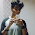## Tuesday, 4 July 2017

### Gaussian Mixture Model (GMM) implementation on Iris data (Python)

Hello there,

In this post, I've implemented unsupervised clustering of Iris dataset using Gaussian mixture models (GMM) in python. A detailed introduction about GMM is available on this Wikipedia page.  The original implementation of the code was done by McDickenson available here in Github-  considering two Gaussian mixture model as inputs. Here, I've modified the code using Iris data as input in 2D.

### Code begins

1. Importing necessary libraries and initial settings

import numpy as np
import pandas as pd
import matplotlib.pyplot as plt
from scipy.stats import norm
import random as rand
from sys import maxint
from sklearn import datasets

2. Importing data from Iris dataset

iris = datasets.load_iris()
X = iris.data[:,:2]
Y = iris.target
Y[:] = Y+1;

Note that I've considered only two dimension of the Iris data which is usually 4 dimensional for better visualisation purpose. iris.target holds the labels for the data and hence Y is nothing but an array of labels

Making a dictionary and dataframe out of the available data

data = {'x':X[:,0],'y':X[:,1], 'label':Y}
df = pd.DataFrame(data=data)

3. Visualising the actual dataset

Before implementing any ML algorithm, it's always good to visualise the date. Let us do this

fig = plt.figure()
plt.scatter(data['x'],data['y'],34,c=Y)

c=Y here is used to identify the label for each data and assign color accordingly

4. Initialization of parameters

Gaussian Mixture models work using Expectation Maximisation algorithm which initially assumes parameters of the model, and using the assumed parameters, it updates the parameters by maximising it by applying to a function. Then it again uses this maximised parameters for the further expectation of the parameters and the cycle continues until convergence.

So, we initially need to input parameters (mean (mu), variance (sig) and the probability that a point can be drawn from the mixture (lambda)). Assuming that we are focused on three clusters and in order to do this, we sample three data points randomly from the input data as the initial means. Choosing some point which is not in the range of the dataset results in unexpected behaviour and you will not see clustering happening. So, the chosen initial means should be in the dataset range.

Choose three random index no's from the length of dataset

k1 = rand.randrange(len(X))
k2 = rand.randrange(len(X))
k3 = rand.randrange(len(X))

Make intial guesses using the index numbers

guess = { 'mu1':[X[k1,0],X[k1,1]],
'sig1':[[1, 0],[0, 1]],
'mu2':[X[k2,0],X[k2,1]],
'sig2':[[2, 0],[0, 1]],
'mu3':[X[k3,0],X[k3,1]],
'sig3':[[0.5, 0],[0, 1]],
'lambda':[0.3,0.3,0.4]
}

Some other initials

shift = maxint
epsilon = 0.01
iters = 0
df_copy = df.copy()

Initially, assign all data points randomly to one of the clusters

df_copy['label']=map(lambda x:x+1, np.random.choice (3,len(df)))

Further

params = pd.DataFrame.from_dict(guess, orient = 'index')
params = params.transpose()

5. Loop until convergence

while shift > epsilon:
iters += 1

Expectation-step

updated_labels = expectation(df_copy.copy(), params)

Maximization-step

updated_parameters = maximization(updated_labels, params.copy())

Updating the shift distance

shift = distance(params, updated_parameters)

Finally, updating the new labels and parameters for the next iteration

df_copy = updated_labels
params = updated_parameters

The function here is used to evaluate whether the distance between points within the cluster is reducing or not. In other words, it's acting like a cost function. If the distance change is very less (less than epsilon threshold) then it's time to stop the algorithm. This function looks like

Function: distance()

def distance(old_params, new_params):
dist = 0
for param in ['mu1','mu2','mu3']:
for i in range(len(old_params)):
dist+=(old_params[param][i]-new_params[param][i])**2
return dist**0.5

The function Expectation assigns labels based on the probability of the data sample being sampled from a Gaussian mixture.

Function: expectation()

def expectation(dataFrame, parameters):
for i in range(dataFrame.shape):
x = dataFrame['x'][i]
y = dataFrame['y'][i]
#assigning the probablilities of each cluster
p_cluster1=prob([x,y],list(parameters['mu1']), list(parameters['sig1']),parameters['lambda'])
p_cluster2=prob([x, y],list(parameters['mu2']), list(parameters['sig2']) parameters['lambda'])
p_cluster3=prob([x, y],list(parameters['mu3']), list(parameters['sig3']),parameters['lambda'])
# Labelling each data according to the probabilities of cluster
if(p_cluster1>=p_cluster2)&(p_cluster1>= p_cluster3):
dataFrame['label'][i] =1
elif(p_cluster2>=p_cluster1)&(p_cluster2>= p_cluster3):
dataFrame['label'][i] = 2
elif(p_cluster3>=p_cluster1)&(p_cluster3>= p_cluster2):
dataFrame['label'][i]=3
else:dataFrame['label'][i]=np.random.choice(3, len(df))+1
return dataFrame

Function prob above is given by

Function: prob()

def prob(val, mu, sig, lam):
p = lam
for i in range(len(val)):
p *= norm.pdf(val[i], mu[i], sig[i][i])
return p

Now, fucntion Maximization looks like

Function: maximization()

def maximization(dataFrame, parameters):
points_assigned_to_cluster1=dataFrame[dataFrame['label']==1]  points_assigned_to_cluster2=dataFrame[dataFrame['label']==2]  points_assigned_to_cluster3=dataFrame[dataFrame['label'] == 3]
percent_assigned_to_cluster1=len(points_assigned_to_cluster1)/float(len(dataFrame))
percent_assigned_to_cluster2=len(points_assigned_to_cluster2)/float(len(dataFrame))
percent_assigned_to_cluster3=1-percent_assigned_to_cluster1-percent_assigned_to_cluster2
parameters['lambda']=[percent_assigned_to_cluster1,percent_assigned_to_cluster2,percent_assigned_to_cluster3 ]
parameters['mu1']=[points_assigned_to_cluster1['x'].mean(),points_assigned_to_cluster1['y'].mean(),None]
parameters['mu2']=[points_assigned_to_cluster2['x'].mean(),points_assigned_to_cluster2['y'].mean(),None]
parameters['mu3']=[points_assigned_to_cluster3['x'].mean(),points_assigned_to_cluster3['y'].mean(),None]
parameters['sig1']= [[points_assigned_to_cluster1['x'].std(),0 ], [0,points_assigned_to_cluster1['y'].std()],None]
parameters['sig2']= [[points_assigned_to_cluster2['x'].std(),0], [0,points_assigned_to_cluster2['y'].std()],None]
parameters['sig3']= [[points_assigned_to_cluster3['x'].std(),0], [0,points_assigned_to_cluster3['y'].std()],None]

return parameters

Now that we have all the functions working, putting it together and running the code will result in the following results

Actual 2D Iris data sample
Three clusters from GMM algorithm

Modifying the same code for two clusters resulted in the following result after just two iteration
Two clusters from GMM algorithm

Hope, this post helped you

You can download the code from Github repository here for free

#### 2 comments:

1.Bhanu, Can I have your mail ID or contact number, I need to ask you something.

2.Hi,
Could you please give an explanation by putting numerical values for a single iteration?

Thanks in advance